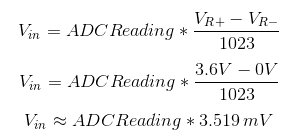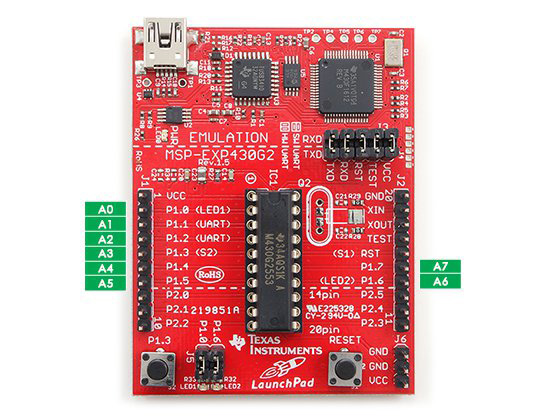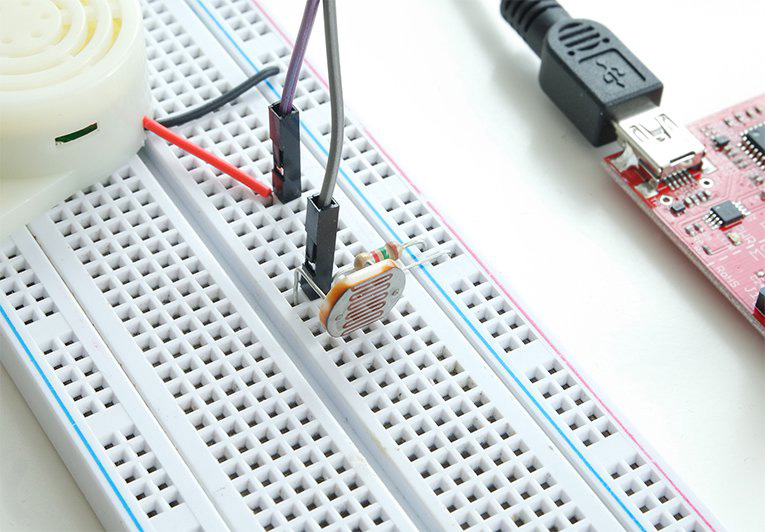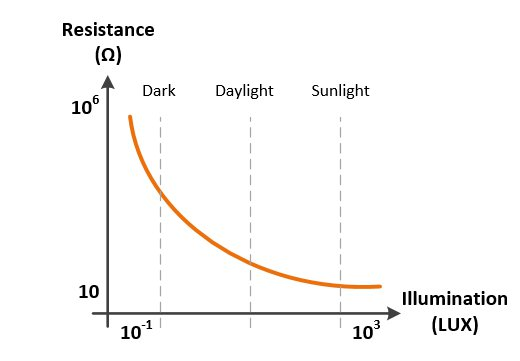The best tools to make your project dreams come true

# Make a Laser Tripwire Alarm with LaunchPadCourtesy of All About Circuits - Before we get started, you will need to understand analog to digital conversion in Launchpad. The LaunchPad MSP430 microcontroller comes with a built-in 10-Bit Analog to Digital Converter (ADC) module that will convert the analog voltage applied to its input into a digital number. In this article you’ll learn how to use LaunchPad’s ADC and make an application developed in EnergiaIDE.

• 10-Bit resolution
• Conversion rate of 200 ksps
• SAR Core (Successive approximation register)
• 8 input channels
• Programmable internal voltage reference
• External voltage reference input
• Programmable sample and hold circuit

The input voltage is converted to a number between 0 and 1023 by the 10-Bit ADC module. These are the lower and the upper voltage limits are expressed as VR and VR-. With the MSP430, the user can select several different reference limits. These can be a combination of supply voltage (VCC) and ground, external reference inputs VREF / VEREF and VREF- / VEREF-, and internal voltage generators 2.5V and 1.5V. If we use the supply voltage VCC (3.6V) and GND as the reference limits, the readings 0 and 1023 will be represented by 0 and 3.6V respectively. Any ADC reading between them can be calculated with the equation below.In Energia, the ADC reference source can be selected using the analogReference(option) function. The options for this function are INTERNAL1V5 (VR = 1.5V, VR- = 0V), DEFAULT (VR = VCC, VR- = 0V), INTERNAL2V5 (VR = 2.5V, VR- = 0V), and EXTERNAL (VR = VREF, VR- = 0V). More options can be found by direct accessing the ADC10CTL0 register.

In order to get a valid conversion result, the voltage applied to an analog input pin must be within the voltage range VR- to VR . The analog input pin’s absolute maximum voltage rating is VCC.

LaunchPad has eight analog input pins of from A0 to A7 which are shown in the image below. Unfortunately, these pins are not marked on LaunchPad’s PCB.In Energia, The analogRead() function is used to read the analog voltage applied to a selected channel and return an integer from 0 to 1023. For this function, the channel number needs to be used  as the input. The selected channel is connected to the ADC module internally with a hardware multiplexer, while the rest of the channels are kept isolated. For example, the command  “adcreading = analogRead(A4);” will read the analog voltage applied to pin P1.4 (A4) and will write the result to the int type variable “adcreading.” Each conversion takes about 100 microseconds.

Noise from the analog signal can distort the conversion result and cause incorrect readings during the analog to digital conversion. Using the oversampling and averaging technique is a good practice to increase the signal to noise ratio (SNR). For example, reading five samples in succession and taking their average will give more accurate results than reading a single sample.

There are some other channels connected internally to the ADC module (see the ADC10CTL1 section of the MSP430x2xx Family User’s Guide for more information). One of those channels is the output of the internal temperature sensor. You can read the temperature sensor data, by using the analogRead(TEMPSENSOR); command.

Making a Laser Tripwire Alarm

You’re now ready to build an application using LaunchPad’s ADC feature. You will need, a light dependent resistor, a laser diode, a resistor, a buzzer, and of course, LaunchPad to make your laser tripwire alarm.A light dependent resistor (LDR) is a semiconductor with a resistor that changes depending on the light intensity on its surface. In a dark environment, the resistance can increase by several mega-ohms; in the light, the resistance can decrease down to a few hundred ohms. The characteristic curve of an LDR is shown below.The LDR will be used as the laser sensor for this application. The resistance of the LDR is about 1-2 kilo-ohms in a room with daylight. When the laser beam hits the LDR’s surface, the resistance decreases down to 100-200 ohms. We will need to know the LDR’s resistance in order to detect whether the “tripwire” is cut. Since LaunchPad’s ADC module can read analog voltage, we should convert this resistance information into voltage. A series resistance is connected to the LDR and the VCC voltage will be applied to the resistor network in order to achieve this. The voltage output of this divider will change Depending on the LDR’s resistance. The application’s schematic can be seen below.

Scheme-it Schematic

When the laser beam hits the LDR’s surface, the resistance is measured at about 200 ohms. In this condition, the on the A0 input’s becomes [3.6V/(1.5K 0.2K)]  * 1.5K = 3.18V. The LDR’s resistance will increase and the A0 input’s voltage will decrease when our “tripwire” is cut. If the A0 input reads less than 3.0V, our “wire” is cut and the alarm should activate.

The Energia code is shown below:

Copy Code
`// the setup routine runs once when you press reset:void setup() { analogRefrence(DEFAULT); // Set VR  = VCC:3.6B, VR- = GND:0V as the upper and the lower limits pinMode(3,OUTPUT); // set the buzzer pin mode}// the loop routine runs over and over again forever:void loop() {  // read the analog voltage at A0  int sensorValue = analogRead(A0);   // convert the ADC reading to voltage  float voltage = sensorValue * (3.6 / 1023);    if (voltage < 3.0) {      // tripwire is cut: activate the buzzer with oscillation    digitalWrite(3,HIGH);     delay(150);    digitalWrite(3,LOW);     delay(100);  }          else {    // tripwire is not cut: de-activate the buzzer     digitalWrite(3,LOW);  }    }`

You can see the laser tripwire alarm in action below!